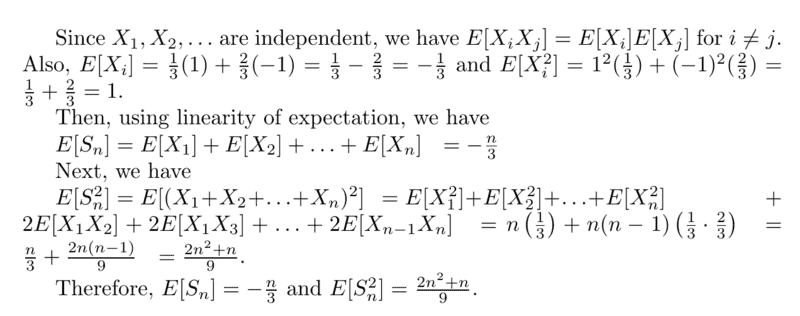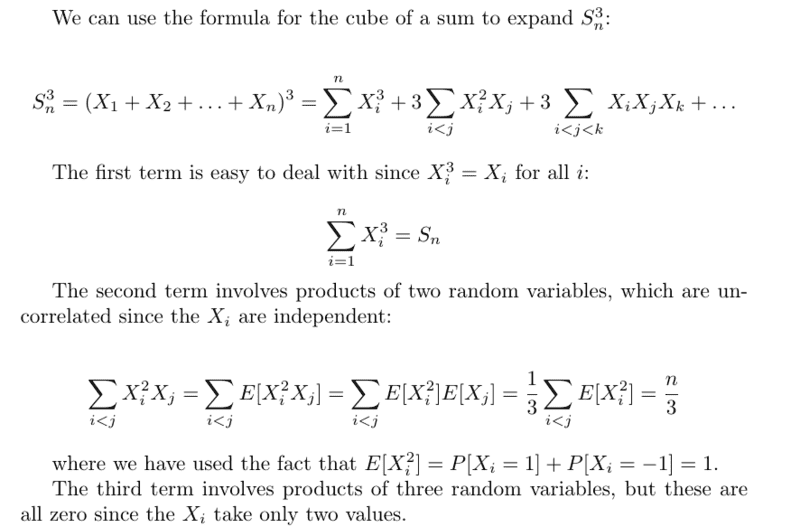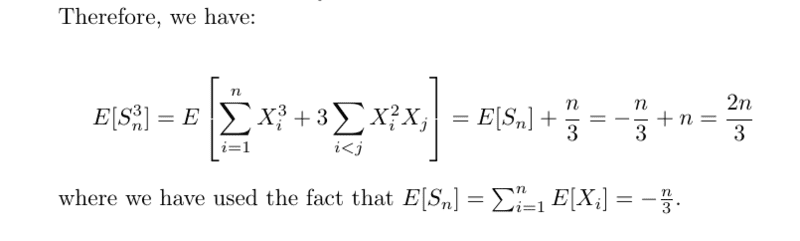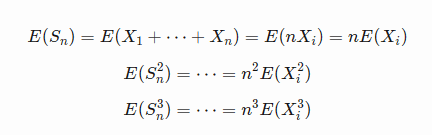# Stochastic Calculus: Conditional Expectation

WMDhamnekar
MHB
Homework Statement:
Suppose ##X_1,X_2 \dots ## are independent random variables with ##\mathbb{P}[X_j= 1] =1- \mathbb{P}[X_j=-1]=\frac13## Let ## S_n = X_1 +\dots +X_n## Find ## \mathbb{E}[S_n], \mathbb{E}[S^2_n], \mathbb{E}[S^3_n]##
Relevant Equations:
Not applicableLast edited:

Homework Helper
Have you tried the simple expedient of substituting some values of n and seeing if you get the right answer? Try n=1 to start.
You are making some careless mistakes
e.g. the expression for E(S2) should be n(1) + n(n-1)(1/9)
Your statement about the product of 3 random variables makes no sense; they are certainly not equal to zero. Each of the 3 random variables may independently take either of the values 1 or -1.

••WMDhamnekar and WWGD
Gold Member
Homework Statement:: Suppose ##X_1,X_2 \dots ## are independent random variables with ##\mathbb{P}[X_j= 1] =1- \mathbb{P}[X_j=-1]=\frac13## Let ## S_n = X_1 +\dots +X_n## Find ## \mathbb{E}[S_n], \mathbb{E}[S^2_n], \mathbb{E}[S^3_n]##
Relevant Equations:: Not applicable

View attachment 323141
View attachment 323142
View attachment 323157
I agree with mjc -- hey, it rhymes!

You can't treat a multinomial ## ( X_1+X_2+....+X_n)^2## as a standard binomial ##( X_1+X_2)^2##. Look up multinomial coefficients.

WMDhamnekar
MHB
Have you tried the simple expedient of substituting some values of n and seeing if you get the right answer? Try n=1 to start.
You are making some careless mistakes
e.g. the expression for E(S2) should be n(1) + n(n-1)(1/9)
Your statement about the product of 3 random variables makes no sense; they are certainly not equal to zero. Each of the 3 random variables may independently take either of the values 1 or -1.
So, taking into consideration your this reply, I correct my amswers as follows:
##\mathbb{E}[S_n]=-\displaystyle\frac{n}{3}, \mathbb{E}[S^2_n]= n +\displaystyle\frac{n(n-1)}{9}, \mathbb{E}[S^3_n ] = -\displaystyle\frac{n}{3}-\frac{n(n-1)}{2} -\displaystyle\frac{n(n-1)}{9}##

Now, are these above answers correct?

Last edited:
WMDhamnekar
MHBHomework Helper
No, they are not. The answer for E(Sn2) is wrong for n = 2, and that for E(Sn3) is wrong for n = 3.

The answer you gave in post #4 for E(Sn2) is right, but the answer for E(Sn3) is not. You didn't show your working, but I suspect the mistake may lie in enumerating the terms of different kinds.
There are n terms of the form Xi3, each of which has expectation -1/3.
There are 3n(n-1) terms of the form Xi2Xj, each of which has expectation -1/3*1.
There are n(n-1)(n-2) terms of the form XiXjXk, each of which has expectation -1/27.
(Check that the total number of terms is n3.)

•WMDhamnekar
•